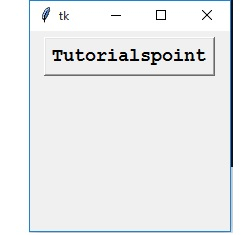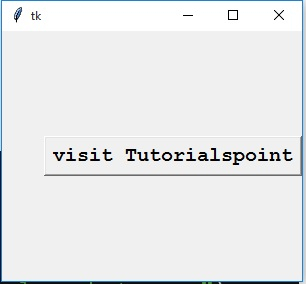# Python - geometry method in Tkinter

Python has capability to create GUI applications using the Tkinter library. The library provides many methods useful for GUI applications. The geometry method is a fundamental one which decides the size, position and some other attributes of the screen layout we are going to create.

## Example - 1

In the below program we create a window of size 22x200 pixels using the geometry method. Then we add a button to it and decide button position in the window using the side and pady options.

## Example

from tkinter import *
base = Tk()
base.geometry('200x200')
stud = Button(base, text = 'Tutorialspoint', font =('Courier',14, 'bold'))
stud.pack(side = TOP, pady = 6)
mainloop()

Running the above code gives us the following result:## Example-2

In this example we create a canvas with a clickable link which will enable us to visit a URL. Again we use the geometry method to create the canvas of the required size.

## Example

import webbrowser
from tkinter import*
def Uniform_Resource_Locator():
url=webbrowser.open_new("http://tutorialspoint.com")
main=Tk()
main.geometry("300x250")
stud=Button(main, text="visit Tutorialspoint", font=('Courier',15,'bold'), command=Uniform_Resource_Locator)stud.pack(side = RIGHT, pady = 6)
main.mainloop()

Running the above code gives us the following resultUpdated on: 23-Dec-2019

4K+ Views